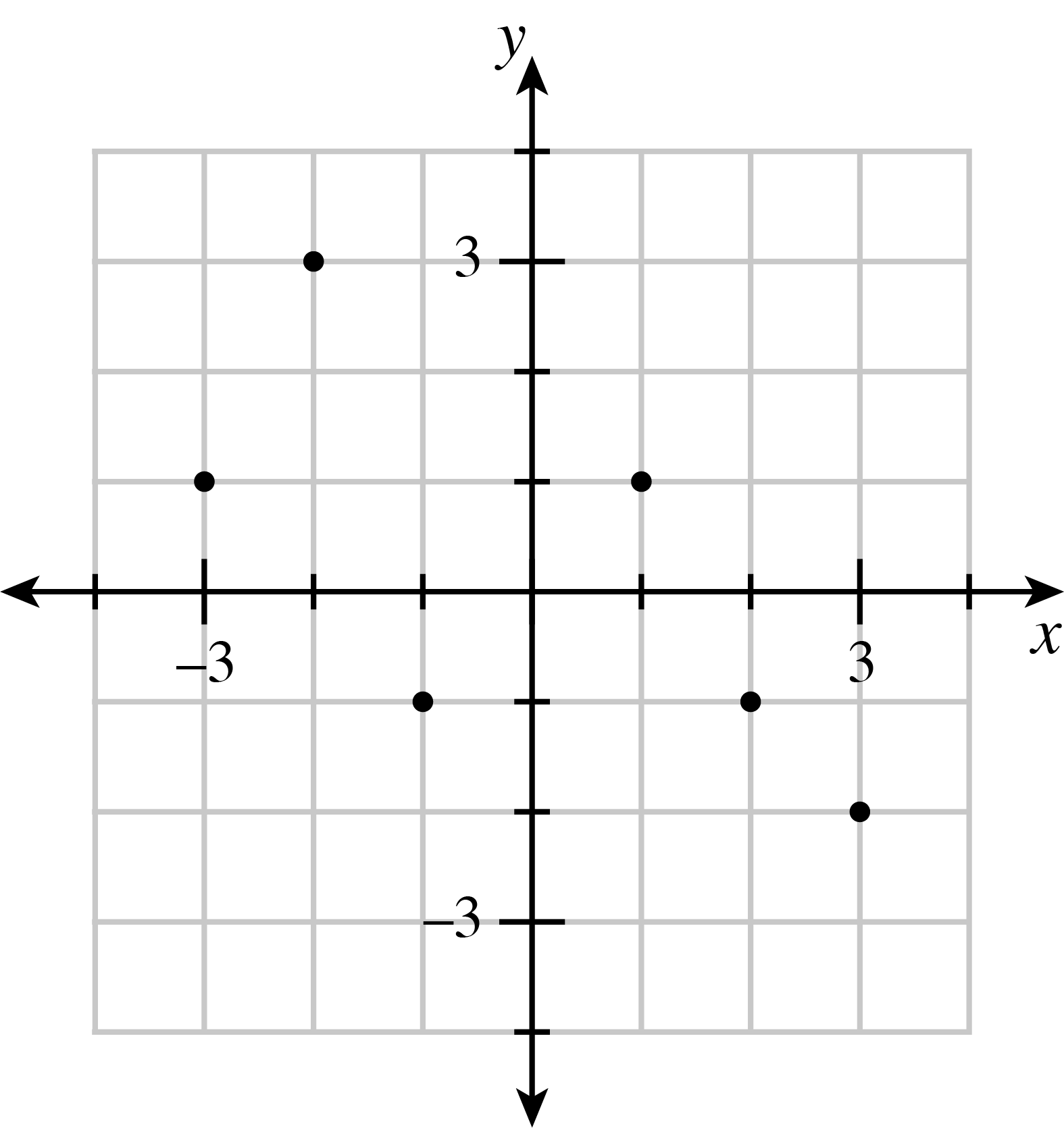### Home > ACC7 > Chapter 1 Unit 1 > Lesson CC3: 1.1.2 > Problem1-16

1-16.Use the graph at right to answer the following questions about quadrants and coordinates of points. Read the Math Notes box in this lesson if you need to review these concepts.

1. What are the coordinates of the two points in Quadrant II?

Match the number on the $x$-coordinate with the number on the $y$-coordinate of the two points in Quadrant II.
$(−3,1)$ and $(−2,3)$
$(2,−1)$ and $(3,−2)$